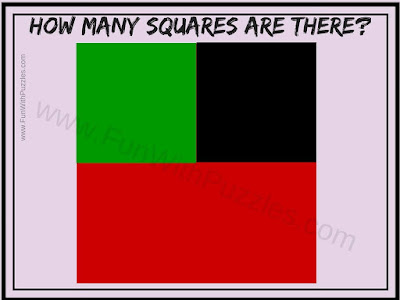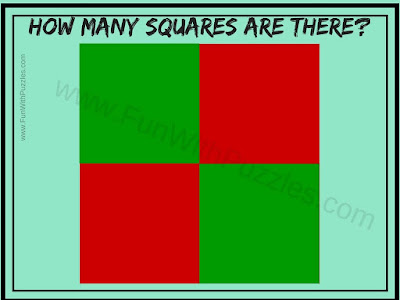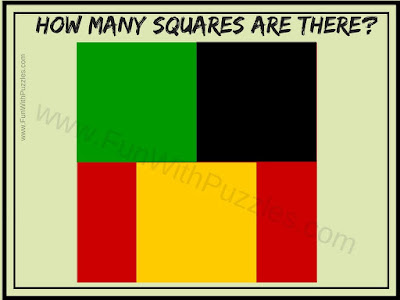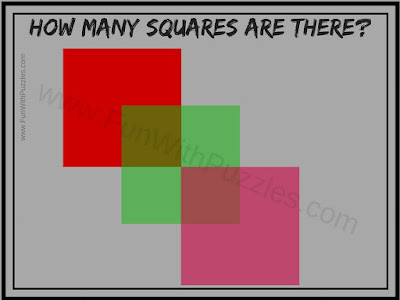Earlier we have published puzzles in which one has to count the number of lines in the given figure. These puzzles were very easy. Later we published the observational puzzles in which one has to count the number of triangles in the given picture puzzles. These puzzles were tougher than the line counting puzzles. Now it's time to add another dimension to counting puzzles. In these puzzles, you have to count the number of squares in each of the given picture puzzle images. These puzzles have been arranged in the order of difficulty level. The first one is very easy and even kids will solve it within no time. However as to move down the number of squares keeps increasing and hence the difficulty level of the puzzles increases.

## How to Solve Count the Squares Picture Puzzles

In these picture puzzles, some square pictures are given. Your challenge in each of the picture puzzle is to count the number of squares. Do remember that every square is a rectangle but not all the rectangles are squares.
Let us start with counting the number of squares in each of the picture puzzle given below1. Can you count the number of Squares in this Picture?2. How many squares can you count in this Picture?3. How many squares are there in this Picture Puzzle?4. Count the total number of squares you see in this picture?5. How many squares can you count in this Picture?

Answer to these counting the number of Squares picture puzzles will be given later. By that time do post your answers in the comment section. Also, check out the similar Picture Puzzles to give a further twist to your mind.

## List of Picture Puzzles and Brain Teasers

1. Picture Puzzles with Answers: Here you can find many different types of picture puzzles to boggle your brain.

2. Odd One Out Picture Puzzles for Teens with Answers: Here you can find medium-level picture puzzles in which your challenge is to find the Odd Picture Out among the given four pictures. If you love Spot the difference picture puzzle then you should definitely try out these puzzles.

3. Intelligent Reading Brain Teasers to Twist your mind: Here are picture puzzles in which either you have to read the given text or you have to find out the hidden letters and numbers. If you think your observational power is very good then you should definitely try out these reading challenges.

4. Brain Wiring Test with Answers: Here are the different types of puzzles. Brain Teasers here include not only Picture Puzzles but also Mathematical and Logical Puzzles. Do try this brain wiring test to check your IQ.